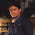## Objective Questions from Opamp

  An ideal OP-AMP is an ideal
A. Current controlled Current source
B. Current controlled Voltage source
C. Voltage controlled Voltage source
D. Voltage controlled Current source

 A 741-Type OP-AMP has a gain-bandwith product of 1MHz. A non-inverting amplifier using this opamp & having a voltage gain of 20db will exhibit -3db bandwidth of
A. 50KHz
B. 100KHz
C. 1000/17KHz
D. 1000/7.07KHz

 An amplifier using an opamp with slew rate SR=1v/sec has a gain of 40db.If this amplifier has to faithfully amplify sinusoidal signals from dc to 20KHz without introducing any slew-rate induced distortion, then the input signal level exceed
A. 795mV
B. 395mV
C. 795mV
D. 39.5mV

 The ideal OP-AMP has the following characteristics
A. Ri=∞,A=∞,R0=0
B. Ri=0,A=∞,R0=0
C. Ri=∞,A=∞,R0=∞
D. Ri=0,A=∞,R0=∞

 The approximate input impedance of the opamp circuit which has Ri=10k, Rf=100k, RL=10k
A. ∞
B. 120k
C. 110k
D. 10k

 An opamp has a slew rate of 5V/ S. the largest sine wave o/p voltage possible at a frequency of 1MHz is
A. 10 V
B. 5 V
C. 5V
D. 5/2 V

 Assume that the op-amp of the fig. is ideal. If Vi is a triangular wave, then V0 will be
A. Square wave
B. Triangular wave
C. Parabolic wave
D. Sine wave

 A differential amplifier is invariably used in the i/p stage of all op-amps. This is done basically to provide the op-amps with a very high
A. CMMR
B. Bandwidth
C. Slew rate
D. Open-loop gain

 A differential amplifier has a differential gain of 20,000. CMMR=80dB. The common mode gain is given by
A. 2
B. 1
C. 1/2
D.0

 In the differential voltage gain & the common mode voltage gain of a differential amplifier are 48db & 2db respectively, then its common mode rejection ratio is
A. 23dB
B. 25dB
C. 46dB
D. 50dB

7:32 AM

#### 7 comments:

1.answer of Q9 wroung. It must be A.2

1.yes it must be 2

2.yes it must be 2

3.2.Yep It is 2 Answer for 10 is also wrong ?

1.Yes, it should be c) i.e. 46 db.
CMRR= Ad/Ac
20 log (CMRR)= 20 log Ad- 20 log Ac=48-2=46 db.

3.